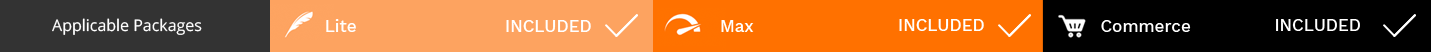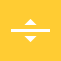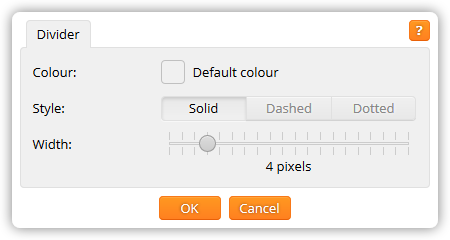The divider component, whose option icon is shown in figure 1, creates a horizontal dividing line.Figure 1. the divider option icon

Dividers can be used to separate content on a page, and are particularly useful for providing additional structure on text-heavy pages.

Editing a divider

Above each divider is a divider bar, as shown in figure 2.Figure 2. a divider bar

Click on the divider bar to open the divider window, as shown in figure 3.Figure 3. the divider window

The divider window lets you change the following settings:

Colour

The colour of the divider.

Style

The style of divider: a solid line, a dashed line, or a dotted line.

Width

The width of the divider, in pixels.

Click OK to apply the changes to the divider. Click Cancel to return to the page without changing the divider.